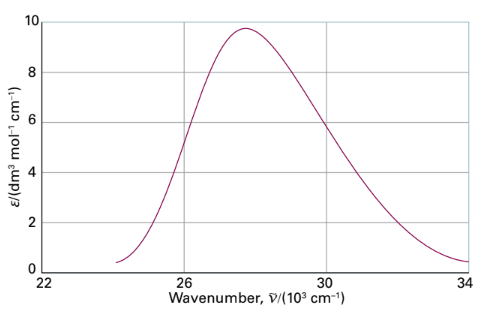×
Get Full Access to Atkins' Physical Chemistry - 11 Edition - Chapter 11a - Problem P11a.6
Get Full Access to Atkins' Physical Chemistry - 11 Edition - Chapter 11a - Problem P11a.6

×ISBN: 9780198769866 2042

## Solution for problem P11A.6 Chapter 11A

Atkins' Physical Chemistry | 11th Edition

• Textbook Solutions
• 2901 Step-by-step solutions solved by professors and subject experts
• Get 24/7 help from StudySoup virtual teaching assistantsAtkins' Physical Chemistry | 11th Edition

4 5 1 418 Reviews
31
3
Problem P11A.6

In many cases it is possible to assume that an absorption band has a Gaussian lineshape (one proportional to $$e^{-x^{2}}$$) centred on the band maximum. Assume such a lineshape, and show that $$\mathcal{A}=\int \varepsilon(\bar{v}) \mathrm{d} \bar{v} \approx 1.0645 \varepsilon_{\max } \Delta \bar{v}_{1 / 2}$$, where $$\Delta \tilde{v}_{1 / 2}$$ is the width at half-height. The absorption spectrum of azoethane $$\left(\mathrm{CH}_{3} \mathrm{CH}_{2} \mathrm{~N}_{2}\right)$$ between 24 000 $$\mathrm{cm}^{-1}$$ and 34 000 $$\mathrm{cm}^{-1}$$ is shown in Fig. 11.2. First, estimate $$\mathcal{A}$$ for the band by assuming that it is Gaussian. Then use mathematical software to fit a polynomial (or a Gaussian) to the absorption band, and integrate the result analytically.Text Transcription:

e^-x^2

A=intvarepsilonvdv1.0645varepsilon_Deltav_1/2

Deltatildev_1/2

CH_3CH_2N_2

cm^-1

A

Step-by-Step Solution:
Step 1 of 3

CHAPTER 7 STUDY NOTES ALKYL HALIDES AND NUCLEOPHILIC SUBSTITUTION - Section 7.1: Introduction to Alkyl Halides o Alkyl halides are organic molecules containing a halogen atom, X, bonded to an sp3 hybridized carbon atom. o Allylic halides have X bonded to the carbon atom adjacent to a carbon-carbon double bond - Section 7.3: Physical Properties of Alkyl Halides o Typically polar so they have small dipole-dipole interactions because of the polar C-X bond. - Section 7.4: Interesting Alkyl Halides o CHCl3  Chloroform or trichloromethane o CCl4  Carbon tetrachloride or tetrachloromethane o Teflon o PVC  Poly(vinyl chloride) o Chlorofluorocarbons - Section 7.5: The Polar Carbon-Halogen Bond o The properties of alkyl halides is what dictates how they react in a reaction o The characteristic reactions of alkyl halides are substitution and elimination  Undergo substitution with nucleophiles  Undergo elimination with Bronsted-Lowry bases - Section 7.6: General Features of Nucleophilic Substitution o Three components are necessary  R: an alkyl R group containing an sp3 hybridized carbon bonded to X  X: an atom called a leaving group which is able to accept the electron density in the C-X bond  Most common leaving groups o Water o N2

Step 2 of 3

Step 3 of 3

##### ISBN: 9780198769866

This textbook survival guide was created for the textbook: Atkins' Physical Chemistry, edition: 11. Since the solution to P11A.6 from 11A chapter was answered, more than 201 students have viewed the full step-by-step answer. The full step-by-step solution to problem: P11A.6 from chapter: 11A was answered by Aimee Notetaker, our top Chemistry solution expert on 04/25/22, 03:45PM. Atkins' Physical Chemistry was written by Aimee Notetaker and is associated to the ISBN: 9780198769866. The answer to “?In many cases it is possible to assume that an absorption band has a Gaussian lineshape (one proportional to $$e^{-x^{2}}$$) centred on the band maximum. Assume such a lineshape, and show that $$\mathcal{A}=\int \varepsilon(\bar{v}) \mathrm{d} \bar{v} \approx 1.0645 \varepsilon_{\max } \Delta \bar{v}_{1 / 2}$$, where $$\Delta \tilde{v}_{1 / 2}$$ is the width at half-height. The absorption spectrum of azoethane $$\left(\mathrm{CH}_{3} \mathrm{CH}_{2} \mathrm{~N}_{2}\right)$$ between 24 000 $$\mathrm{cm}^{-1}$$ and 34 000 $$\mathrm{cm}^{-1}$$ is shown in Fig. 11.2. First, estimate $$\mathcal{A}$$ for the band by assuming that it is Gaussian. Then use mathematical software to fit a polynomial (or a Gaussian) to the absorption band, and integrate the result analytically. Text Transcription:e^-x^2A=intvarepsilonvdv1.0645varepsilon_Deltav_1/2Deltatildev_1/2CH_3CH_2N_2cm^-1A” is broken down into a number of easy to follow steps, and 109 words. This full solution covers the following key subjects: . This expansive textbook survival guide covers 327 chapters, and 1120 solutions.

## Discover and learn what students are asking

Chemistry: The Central Science : Molecular Geometry and Bonding Theories
?How does a trigonal pyramid differ from a tetrahedron so far as molecular geometry is concerned?

Unlock Textbook Solution